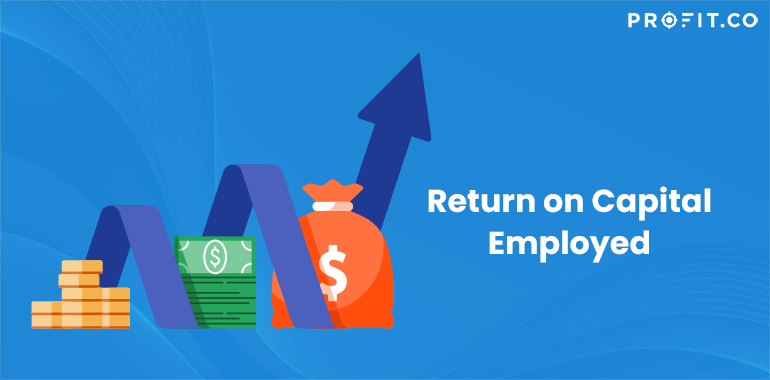Category: Finance KPIs.

To better understand what the return on capital employed means, first we need to understand what the capital employed is. By employing capital, you will certainly make a profit, right? So, this is the amount of capital used for making profits. Back to the return on capital employed, this term or ratio means the amount of money that you gain from the capital employed.

To get a better understanding of what the capital employed really means, just add the fixed assets to the working capital and you will get the capital employed. But calculating the return on capital employed is another piece of calculation. So, read on to find out more.

How to calculate the Return on Capital Employed?

The formula is quite simple. You can divide the net operating profit to the employed capital. So, the formula should look like this:

Return on Capital Employed
=
Net Operating ProfitNet Operating Profit / Employed Capital

As per above statements regarding the capital employed, if you don’t have the Capital Employed, you might as well calculate it by adding the fixed assets to the working capital. This formula will look something like this:

Return on Capital Employed
=
Net Operating Profit(Fixed Assets + Working Capital)

Believe it or not, there is a third way of calculation the Employed Capital. This can be calculated by simply subtracting the Total Assets from the Current Liabilities. Then, you’ll have another formula similar to the main one. So, the third formula should look like this:

Return on Capital Employed
=
Net Operating Profit(Total Assets – Current Liabilities)

With so many ways of calculating the return on capital employed, you can’t go wrong. By doing the math right, you can make sure that you have the same result on any formula.

Analyzing the return on Capital Employed Formula

Although you can use the return on equity to calculate the same thing, analysts prefer the return on capital employed because it shows the profitability of a company for a very long time. The results can be interpreted as follows: a higher return on capital employed means that the company is successful in terms of capital employed. The return should always be higher than the rate at which the company borrows money for assets. If the return is lower, then the company loses money. A return of 10 percent for a borrowing of 15 percent means that the company is also losing money.

A useful tip is to keep the assets level low for the return to be higher. The more assets you buy, the less return you’ll get. For a company to be successful, the return should be twice the amount of the money borrowed to get assets.

As you can see, the return on capital employed has lots of formulas that you can use and if you get the same result with every formula, then you got yourself a win. However, if you miss some important aspects of the calculation and you don’t organize your assets correctly, you can get yourself in a loss.

## Related Articles

• ### Sharpe Ratio

In order to be sound in your financial goals, you need to have a great source of knowledge on every... Read more